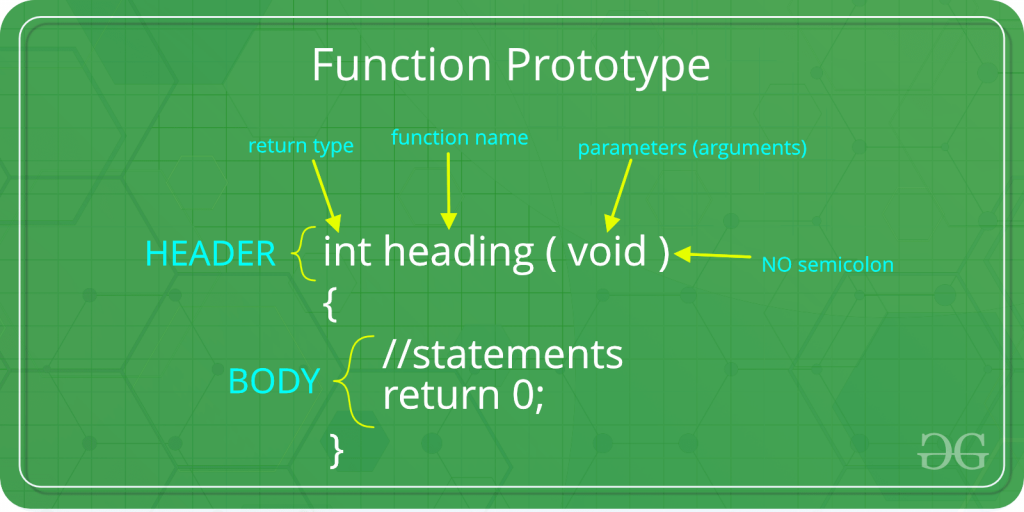# B.Tech. (SEM-II) THEORY EXAMINATION 2017-18 COMPUTER SYSTEM & PROGRAMMING IN C

Time: 3hrs
Total Marks: 70

Note:-

• There are three sections. Section A carries 20 marks, Section B carries 30 marks and Section C carries 50 marks.
• Attempt all questions. Marks are indicated against each question.
• Assume suitable data wherever necessary.

# Section – A

1. Attempt all parts: (2*7 = 14)

1. What is token in ‘C’ language?

A token is the smallest element of a program that is meaningful to the compiler. Tokens can be classified as follows:

1. Keywords
2. Identifiers
3. Constants
4. Strings
5. Special Symbols
6. Operators
2. What do you mean by formatted output in C language? Explain with example.

Formatted Output means changing the output pattern in a C language as per the specifications. It is done using Format Specifiers in C. Format specifier is used during input and output. It is a way to tell the compiler what type of data is in a variable during taking input using scanf() or printing using printf(). Some examples are %c, %d, %f, etc.

Examples:

 `#include ` `int` `main() ` `{ ` `    ``int` `x = 45, y = 90; ` `    ``char` `ch = ``'A'``; ` `    ``printf``(``"%c\n"``, ch); ` `    ``printf``(``"%d\n"``, x); ` `    ``printf``(``"%i\n"``, x); ` `    ``return` `0; ` `} `

Output:

```A
45
45
```
3. What is the use of fseek( ) function in files. Write its syntax?

fseek() is used to move file pointer associated with a given file to a specific position.
Syntax:

`int fseek(FILE *pointer, long int offset, int position)`

Parameters: This method accepts following parameters:

• pointer: pointer to a FILE object that identifies the stream.
• offset: number of bytes to offset from position
• position: position from where offset is added.
position defines the point with respect to which the file pointer needs to be moved. It has three values:
SEEK_END : It denotes end of the file.
SEEK_SET : It denotes starting of the file.
SEEK_CUR : It denotes file pointer’s current position.

Return Value: This method returns zero if successful, or else it returns a non-zero value

4. Write down the output of the following.

 `main() ` `{ ` `    ``int` `i = 1; ` `    ``for` `(;;) { ` `        ``printf``(“% d”, i); ` `        ``if` `(i = = 7) ` `            ``break``; ` `    ``} ` `} `

Output:

`111111......infinite times`
5. Explain function prototype? Why is it required?

Function prototype tells the compiler about the number of parameters function takes, data-types of parameters and return type of function. By using this information, compiler cross checks function parameters and their data-type with function definition and function call. If we ignore the function prototype, the program may compile with a warning and may work properly. But sometimes, it will give strange output and it is very hard to find such programming mistakes.The Function prototype serves the following purposes –

1) It tells the return type of the data that the function will return.
2) It tells the number of arguments passed to the function.
3) It tells the data types of each of the passed arguments.
4) Also, it tells the order in which the arguments are passed to the function.

Therefore essentially, function prototype specifies the input/output interface to the function i.e. what to give to the function and what to expect from the function.

Prototype of a function is also called signature of the function.

6. What are subscripts? How are they specified?

The definition of [] subscriptok operator operator in C, according to (C99, 6.5.2.1p2), is that:

` E1[E2] is identical to (*((E1)+(E2)))`

Compilers use pointer arithmetic internally to access array elements. And because of the conversion rules that apply to the binary + operator, if E1 is an array object (equivalently, a pointer to the initial element of an array object) and E2 is an integer, E1[E2] designates the E2-th element of E1 (counting from zero).

Therefore, a[b] is defined as :

```a[b] == *(a + b)
```

So will evaluate to

```a == *(a + 8)
```

Here, a is a pointer to the first element of the array and a is the value of an elements which is 8 elements further from a, which is the same as *(a + 8).

7. Write the use of putchar() and getchar().

getchar():
The difference between getc() and getchar() is getc() can read from any input stream, but getchar() reads from standard input. So getchar() is equivalent to getc(stdin).

Syntax:

`int getchar(void); `

Example:

 `// Example for getchar() in C ` `#include ` `int` `main() ` `{ ` `    ``printf``(``"%c"``, ``getchar``()); ` `    ``return` `0; ` `} `

Output:

```Input: g
Output: g ```

putchar(): The putchar(int char) method in C is used to write a character, of unsigned char type, to stdout. This character is passed as the parameter to this method.

Syntax:

`int putchar(int char)`

Parameters: This method accepts a mandatory parameter char which is the character to be written to stdout.

Return Value: This function returns the character written on the stdout as an unsigned char. It also returns EOF when some error occurs.

Below examples illustrate the use of putchar() method:

Example 1:

 `// C program to demonstrate putchar() method ` ` `  `#include ` ` `  `int` `main() ` `{ ` ` `  `    ``// Get the character to be written ` `    ``char` `ch = ``'G'``; ` ` `  `    ``// Write the Character to stdout ` `    ``putchar``(ch); ` ` `  `    ``return` `(0); ` `} `

Output:

```G
```

My Personal Notes arrow_drop_upCheck out this Author's contributed articles.

If you like GeeksforGeeks and would like to contribute, you can also write an article using contribute.geeksforgeeks.org or mail your article to contribute@geeksforgeeks.org. See your article appearing on the GeeksforGeeks main page and help other Geeks.

Please Improve this article if you find anything incorrect by clicking on the "Improve Article" button below.

Article Tags :

Be the First to upvote.

Please write to us at contribute@geeksforgeeks.org to report any issue with the above content.